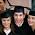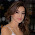## Monday, August 15, 2016

### Middle School Math Solutions – Polynomials Calculator, Adding Polynomials

A polynomial is an expression of two or more algebraic terms, often having different exponents. Adding polynomials is pretty simple. The only tricky part is that there are different terms. The key is to add the like terms. Like terms are terms that have the same variable with the same exponent, even if they have different coefficients. There are three steps to adding polynomials:
1. Remove parentheses - only include this step if the polynomials are in parentheses
2. Group like terms - put together and order like terms (terms with the same variable and same exponent)
3. Add like terms - add the coefficients of the like terms

Let’s check out some examples step by step.

(x^2+2x-1)+(2x^2-3x+6)
1. Remove parentheses
x^2+2x-1+2x^2-3x+6
2. Group like terms
x^2+2x^2+2x-3x-1+6
When I group like terms, I like to group and put the terms in order from the largest exponent to the smallest exponent.

3. Add like terms
3x^2+2x-3x-1+6
3x^2-x-1+6
3x^2-x+5

(2x^3+4x+7)+(2x^2+5x-6)
1. Remove parentheses
2x^3+4x+7+2x^2+5x-6
2. Group like terms
2x^3+2x^2+4x+5x+7-6
3. Add like terms
2x^3+2x^2+9x+1

4x+(4x-2)+(x^2-3)
1. Remove parentheses
4x+4x-2+x^2-3
2. Group like terms
x^2+4x+4x-2-3
3. Add like terms
x^2+8x-2-3
x^2+8x-5

Adding polynomials is pretty simple and requires just a few steps. The trickiest part about adding polynomials is making sure that you pay attention the exponents. It is easy to overlook and confuse a 2 for a 3. For more practice on this topic, check out Symbolab’s Practice.

Until next time,

Leah

1.Thanks for sharing.I found a lot of interesting information here. A really good post, very thankful and hopeful that you will write many more posts like this one.
https://kodi.software/
https://plex.software/
https://luckypatcher.pro/

2.Middle school math solution is here. Students feel trouble in the subject of math that custom papers reviews subject is very difficult for them. Because they want to remove that difficulty which is good for all of students.

3.I am going to write an article on middle School math solutions for Paper Writers and I want to know what is Polynomials Calculator could?

4.I've heard you are looking for an essay writing guide. SO I brought you this https://writemyessay4me.org/blog/illustration-essay.